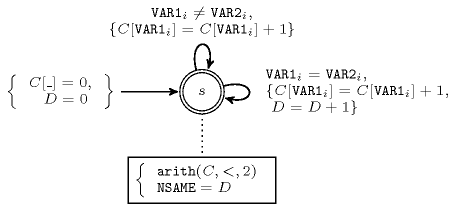## 5.21. alldifferent_same_value

Origin
Constraint

$\mathrm{𝚊𝚕𝚕𝚍𝚒𝚏𝚏𝚎𝚛𝚎𝚗𝚝}_\mathrm{𝚜𝚊𝚖𝚎}_\mathrm{𝚟𝚊𝚕𝚞𝚎}\left(\mathrm{𝙽𝚂𝙰𝙼𝙴},\mathrm{𝚅𝙰𝚁𝙸𝙰𝙱𝙻𝙴𝚂}\mathtt{1},\mathrm{𝚅𝙰𝚁𝙸𝙰𝙱𝙻𝙴𝚂}\mathtt{2}\right)$

Synonyms

$\mathrm{𝚊𝚕𝚕𝚍𝚒𝚏𝚏}_\mathrm{𝚜𝚊𝚖𝚎}_\mathrm{𝚟𝚊𝚕𝚞𝚎}$, $\mathrm{𝚊𝚕𝚕𝚍𝚒𝚜𝚝𝚒𝚗𝚌𝚝}_\mathrm{𝚜𝚊𝚖𝚎}_\mathrm{𝚟𝚊𝚕𝚞𝚎}$.

Arguments
 $\mathrm{𝙽𝚂𝙰𝙼𝙴}$ $\mathrm{𝚍𝚟𝚊𝚛}$ $\mathrm{𝚅𝙰𝚁𝙸𝙰𝙱𝙻𝙴𝚂}\mathtt{1}$ $\mathrm{𝚌𝚘𝚕𝚕𝚎𝚌𝚝𝚒𝚘𝚗}\left(\mathrm{𝚟𝚊𝚛}-\mathrm{𝚍𝚟𝚊𝚛}\right)$ $\mathrm{𝚅𝙰𝚁𝙸𝙰𝙱𝙻𝙴𝚂}\mathtt{2}$ $\mathrm{𝚌𝚘𝚕𝚕𝚎𝚌𝚝𝚒𝚘𝚗}\left(\mathrm{𝚟𝚊𝚛}-\mathrm{𝚍𝚟𝚊𝚛}\right)$
Restrictions
 $\mathrm{𝙽𝚂𝙰𝙼𝙴}\ge 0$ $\mathrm{𝙽𝚂𝙰𝙼𝙴}\le |\mathrm{𝚅𝙰𝚁𝙸𝙰𝙱𝙻𝙴𝚂}\mathtt{1}|$ $|\mathrm{𝚅𝙰𝚁𝙸𝙰𝙱𝙻𝙴𝚂}\mathtt{1}|=|\mathrm{𝚅𝙰𝚁𝙸𝙰𝙱𝙻𝙴𝚂}\mathtt{2}|$ $\mathrm{𝚛𝚎𝚚𝚞𝚒𝚛𝚎𝚍}$$\left(\mathrm{𝚅𝙰𝚁𝙸𝙰𝙱𝙻𝙴𝚂}\mathtt{1},\mathrm{𝚟𝚊𝚛}\right)$ $\mathrm{𝚛𝚎𝚚𝚞𝚒𝚛𝚎𝚍}$$\left(\mathrm{𝚅𝙰𝚁𝙸𝙰𝙱𝙻𝙴𝚂}\mathtt{2},\mathrm{𝚟𝚊𝚛}\right)$
Purpose

All the values assigned to the variables of the collection $\mathrm{𝚅𝙰𝚁𝙸𝙰𝙱𝙻𝙴𝚂}\mathtt{1}$ are pairwise distinct. $\mathrm{𝙽𝚂𝙰𝙼𝙴}$ is equal to number of constraints of the form $\mathrm{𝚅𝙰𝚁𝙸𝙰𝙱𝙻𝙴𝚂}\mathtt{1}\left[i\right].\mathrm{𝚟𝚊𝚛}=\mathrm{𝚅𝙰𝚁𝙸𝙰𝙱𝙻𝙴𝚂}\mathtt{2}\left[i\right].\mathrm{𝚟𝚊𝚛}\left(1\le i\le |\mathrm{𝚅𝙰𝚁𝙸𝙰𝙱𝙻𝙴𝚂}\mathtt{1}|\right)$ that hold.

Example
$\left(2,〈7,3,1,5〉,〈1,3,1,7〉\right)$

The $\mathrm{𝚊𝚕𝚕𝚍𝚒𝚏𝚏𝚎𝚛𝚎𝚗𝚝}_\mathrm{𝚜𝚊𝚖𝚎}_\mathrm{𝚟𝚊𝚕𝚞𝚎}$ constraint holds since:

• All the values 7, 3, 1 and 5 are distinct,

• Among the four expressions $7=1$, $3=3$, $1=1$ and $5=7$ exactly 2 conditions hold.

Typical
 $\mathrm{𝙽𝚂𝙰𝙼𝙴}<|\mathrm{𝚅𝙰𝚁𝙸𝙰𝙱𝙻𝙴𝚂}\mathtt{1}|$ $|\mathrm{𝚅𝙰𝚁𝙸𝙰𝙱𝙻𝙴𝚂}\mathtt{1}|>2$
Symmetries
• Items of $\mathrm{𝚅𝙰𝚁𝙸𝙰𝙱𝙻𝙴𝚂}\mathtt{1}$ and $\mathrm{𝚅𝙰𝚁𝙸𝙰𝙱𝙻𝙴𝚂}\mathtt{2}$ are permutable (same permutation used).

• All occurrences of two distinct values in $\mathrm{𝚅𝙰𝚁𝙸𝙰𝙱𝙻𝙴𝚂}\mathtt{1}.\mathrm{𝚟𝚊𝚛}$ or $\mathrm{𝚅𝙰𝚁𝙸𝙰𝙱𝙻𝙴𝚂}\mathtt{2}.\mathrm{𝚟𝚊𝚛}$ can be swapped; all occurrences of a value in $\mathrm{𝚅𝙰𝚁𝙸𝙰𝙱𝙻𝙴𝚂}\mathtt{1}.\mathrm{𝚟𝚊𝚛}$ or $\mathrm{𝚅𝙰𝚁𝙸𝙰𝙱𝙻𝙴𝚂}\mathtt{2}.\mathrm{𝚟𝚊𝚛}$ can be renamed to any unused value.

Arg. properties

Functional dependency: $\mathrm{𝙽𝚂𝙰𝙼𝙴}$ determined by $\mathrm{𝚅𝙰𝚁𝙸𝙰𝙱𝙻𝙴𝚂}\mathtt{1}$ and $\mathrm{𝚅𝙰𝚁𝙸𝙰𝙱𝙻𝙴𝚂}\mathtt{2}$.

Usage

When all variables of the second collection are initially bound to distinct values the $\mathrm{𝚊𝚕𝚕𝚍𝚒𝚏𝚏𝚎𝚛𝚎𝚗𝚝}_\mathrm{𝚜𝚊𝚖𝚎}_\mathrm{𝚟𝚊𝚕𝚞𝚎}$ constraint can be explained in the following way:

• We interpret the variables of the second collection as the previous solution to a problem where all variables have to be distinct.

• We interpret the variables of the first collection as the current solution to find, where all variables should again be pairwise distinct.

The variable $\mathrm{𝙽𝚂𝙰𝙼𝙴}$ measures the $\mathrm{𝚍𝚒𝚜𝚝𝚊𝚗𝚌𝚎}$ of the current solution from the previous solution. This corresponds to the number of variables of $\mathrm{𝚅𝙰𝚁𝙸𝙰𝙱𝙻𝙴𝚂}\mathtt{2}$ that are assigned to the same previous value.

Keywords
Cond. implications

$\mathrm{𝚊𝚕𝚕𝚍𝚒𝚏𝚏𝚎𝚛𝚎𝚗𝚝}_\mathrm{𝚜𝚊𝚖𝚎}_\mathrm{𝚟𝚊𝚕𝚞𝚎}\left(\mathrm{𝙽𝚂𝙰𝙼𝙴},\mathrm{𝚅𝙰𝚁𝙸𝙰𝙱𝙻𝙴𝚂}\mathtt{1},\mathrm{𝚅𝙰𝚁𝙸𝙰𝙱𝙻𝙴𝚂}\mathtt{2}\right)$

with  $2*\mathrm{𝙽𝚂𝙰𝙼𝙴}=|\mathrm{𝚅𝙰𝚁𝙸𝙰𝙱𝙻𝙴𝚂}\mathtt{1}|$

implies $\mathrm{𝚍𝚒𝚏𝚏𝚎𝚛}_\mathrm{𝚏𝚛𝚘𝚖}_\mathrm{𝚎𝚡𝚊𝚌𝚝𝚕𝚢}_𝚔_\mathrm{𝚙𝚘𝚜}$$\left(𝙺,\mathrm{𝚅𝙴𝙲𝚃𝙾𝚁}\mathtt{1},\mathrm{𝚅𝙴𝙲𝚃𝙾𝚁}\mathtt{2}\right)$.

Arc input(s)

$\mathrm{𝚅𝙰𝚁𝙸𝙰𝙱𝙻𝙴𝚂}\mathtt{1}$ $\mathrm{𝚅𝙰𝚁𝙸𝙰𝙱𝙻𝙴𝚂}\mathtt{2}$

Arc generator
$\mathrm{𝑃𝑅𝑂𝐷𝑈𝐶𝑇}$$\left($$\mathrm{𝐶𝐿𝐼𝑄𝑈𝐸}$$,$$\mathrm{𝐿𝑂𝑂𝑃}$$,=\right)↦\mathrm{𝚌𝚘𝚕𝚕𝚎𝚌𝚝𝚒𝚘𝚗}\left(\mathrm{𝚟𝚊𝚛𝚒𝚊𝚋𝚕𝚎𝚜}\mathtt{1},\mathrm{𝚟𝚊𝚛𝚒𝚊𝚋𝚕𝚎𝚜}\mathtt{2}\right)$

Arc arity
Arc constraint(s)
$\mathrm{𝚟𝚊𝚛𝚒𝚊𝚋𝚕𝚎𝚜}\mathtt{1}.\mathrm{𝚟𝚊𝚛}=\mathrm{𝚟𝚊𝚛𝚒𝚊𝚋𝚕𝚎𝚜}\mathtt{2}.\mathrm{𝚟𝚊𝚛}$
Graph property(ies)
 $•$$\mathrm{𝐌𝐀𝐗}_\mathrm{𝐍𝐒𝐂𝐂}$$\le 1$ $•$$\mathrm{𝐍𝐀𝐑𝐂}_\mathrm{𝐍𝐎}_\mathrm{𝐋𝐎𝐎𝐏}$$=\mathrm{𝙽𝚂𝙰𝙼𝙴}$

Graph model

The arc generator $\mathrm{𝑃𝑅𝑂𝐷𝑈𝐶𝑇}$$\left($$\mathrm{𝐶𝐿𝐼𝑄𝑈𝐸}$$,$$\mathrm{𝐿𝑂𝑂𝑃}$$,=\right)$ is used in order to generate all the arcs of the initial graph:

Part (A) of Figure 5.21.1 gives the initial graph associated with the Example slot. Variables of collection $\mathrm{𝚅𝙰𝚁𝙸𝙰𝙱𝙻𝙴𝚂}\mathtt{1}$ are coloured, while variables of collection $\mathrm{𝚅𝙰𝚁𝙸𝙰𝙱𝙻𝙴𝚂}\mathtt{2}$ are kept in white. Part (B) represents the final graph associated with the Example slot. In this graph each vertex constitutes a strongly connected component and the number of arcs that do not correspond to a loop is equal to 2 (i.e., $\mathrm{𝙽𝚂𝙰𝙼𝙴}$).

##### Figure 5.21.1. (A) Initial and (B) final graph of the $\mathrm{𝚊𝚕𝚕𝚍𝚒𝚏𝚏𝚎𝚛𝚎𝚗𝚝}_\mathrm{𝚜𝚊𝚖𝚎}_\mathrm{𝚟𝚊𝚕𝚞𝚎}$$\left(2,〈{U}_{1},{U}_{2},{U}_{3},{U}_{4}〉$, $〈{V}_{1},{V}_{2},{V}_{3},{V}_{4}〉\right)$ constraint with ${U}_{1}=7$, ${U}_{2}=3$, ${U}_{3}=1$, ${U}_{4}=5$ and ${V}_{1}=1$, ${V}_{2}=3$, ${V}_{3}=1$, ${V}_{4}=7$ (in Part (B) arcs in red correspond to the arcs counted by the argument $\mathrm{𝙽𝚂𝙰𝙼𝙴}$)Automaton

Figure 5.21.2 depicts the automaton associated with the $\mathrm{𝚊𝚕𝚕𝚍𝚒𝚏𝚏𝚎𝚛𝚎𝚗𝚝}_\mathrm{𝚜𝚊𝚖𝚎}_\mathrm{𝚟𝚊𝚕𝚞𝚎}$ constraint. Let $\mathrm{𝚅𝙰𝚁}{\mathtt{1}}_{i}$ and $\mathrm{𝚅𝙰𝚁}{\mathtt{2}}_{i}$ respectively denote the ${i}^{th}$ variables of the $\mathrm{𝚅𝙰𝚁𝙸𝙰𝙱𝙻𝙴𝚂}\mathtt{1}$ and $\mathrm{𝚅𝙰𝚁𝙸𝙰𝙱𝙻𝙴𝚂}\mathtt{2}$ collections. To each pair of variables $\left(\mathrm{𝚅𝙰𝚁}{\mathtt{1}}_{i},\mathrm{𝚅𝙰𝚁}{\mathtt{2}}_{i}\right)$ corresponds a signature variable ${𝚂}_{i}$. The following signature constraint links $\mathrm{𝚅𝙰𝚁}{\mathtt{1}}_{i}$, $\mathrm{𝚅𝙰𝚁}{\mathtt{2}}_{i}$ and ${𝚂}_{i}$: $\mathrm{𝚅𝙰𝚁}{\mathtt{1}}_{i}=\mathrm{𝚅𝙰𝚁}{\mathtt{2}}_{i}⇔{𝚂}_{i}$.

##### Figure 5.21.2. Automaton of the $\mathrm{𝚊𝚕𝚕𝚍𝚒𝚏𝚏𝚎𝚛𝚎𝚗𝚝}_\mathrm{𝚜𝚊𝚖𝚎}_\mathrm{𝚟𝚊𝚕𝚞𝚎}$ constraint